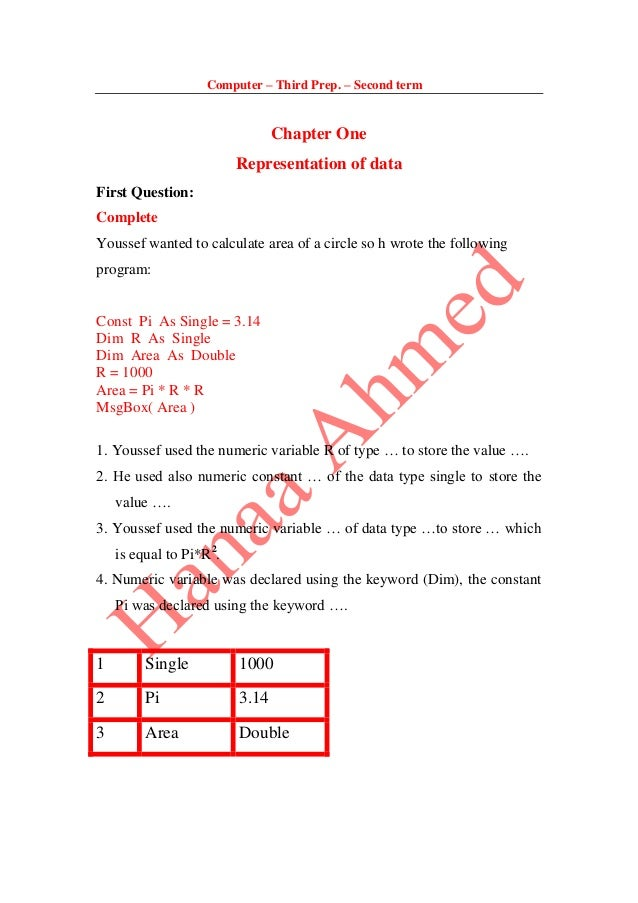Successfully reported this slideshow.
We use your LinkedIn profile and activity data to personalize ads and to show you more relevant ads. You can change your ad preferences anytime.Upcoming SlideShare
Loading in …5
×

# Computer

أسئلة عامة على الفصل الأول من الأعوام السابقة من الوزارة والأمتحانات السابقة مع المنهج الجديد للغات
من إعداد/ هناء أحمد محمد إبراهيم

• Full Name
Comment goes here.

Are you sure you want to Yes No
Your message goes here• Be the first to comment

• Be the first to like this

### Computer

1. 1. Computer – Third Prep. – Second term Chapter One Representation of data First Question: Complete Youssef wanted to calculate area of a circle so h wrote the following program: Const Pi As Single = 3.14 Dim R As Single Dim Area As Double R = 1000 Area = Pi * R * R MsgBox( Area ) 1. Youssef used the numeric variable R of type … to store the value …. 2. He used also numeric constant … of the data type single to store the value …. 3. Youssef used the numeric variable … of data type …to store … which is equal to Pi*R2 . 4. Numeric variable was declared using the keyword (Dim), the constant Pi was declared using the keyword …. 1 Single 1000 2 Pi 3.14 3 Area Double
2. 2. Second question: Choose the correct answer: (Dim, Const, String, Single) 1. Khaled wanted to calculate total grade of a student so he declared numeric variables using the word… 2. He used a variable of data type …. To store student name. 3. He used a variable of data type… to store the total grade. 1 2 3 Dim String Single
3. 3. Question three: Choose the correct Answer: 1. The numeric variable that stores only whole numbers from 0 – 255 is of data type (Byte – Integer – String) 2. A variable of type (Boolean – Integer – String) stores the values truefalse. 3. To store a digit with decimal points(fractions) we use a variable of data type (Integer – Short – Single). 4. To store whole numbers only we use a variable of data type (Single – Double Integer). 5. One of the variable naming rules is that the name has ( to start with 9Digit – Letter – Any symbol). 6. The word (Dim – Const – End) is used to declare variables. 7. The word (Dim – Const – End) is used to declare constants in a program. 8. The command (Const/As – Din/As – Try/Catch) to discover program errors and to deal with it. 9. On executing the equation (4*3+2), the result will be (9 - 20 - 14). 10.The result of the arithmetic operation (2^3+1) is (10 – 16 – 9). 1 2 3 4 5 6 7 8 9 10 Byte Boolean Single Integer Letter Dim Const TryCatch 14 9
4. 4. Fourth Question: Put () or (X): 1. Data stores that can change their values during program execution are called variables ( ) 2. Data stores that can change values during program execution are called constants ( ). 3. Variable of type integer can store whole numbers from 0 to 255 ( ). 4. Variables of data type byte stores whole numbers from 0 to 255 ( ). 5. Boolean variables stores the values “true” or “false” ( ). 6. To store fractional numeric values we use types Integer or Short ( ). 7. To store numeric values with fractions we use the data types Double or Single ( ). 8. One of the variable naming rules is to start the name with a letter ( ). 9. The words (Dim, Integer or Double) can be used as variable names ( ). 10.The word “Dim” is used to declare variables inside the program ( ). 11.The word “Const” is used to declare constants inside the program ( ). 12.Commands (Try/Catch) are used to discover and deal with program errors ( ). 13.From Precedence rules is Addition precedes division on program execution ( ). 14.From Precedence operation is that multiplication operation precedes subtraction operation on program execution ( ). 15.Executing Brackets precedes other operation on program execution ( ). 1 2 3 4 5 6 7 8 9 10 11 12 13 14 15  x x   x   x  x  x  